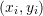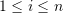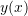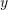### Curve Fitting: Introduction

Given a set of datawith, curve fitting revolves around finding a mathematical model that can describe the relationshipsuch that the prediction of the mathematical model would match, as closely as possible, the given data. There are two advantages to finding an appropriate mathematical model with a good fit. The first advantage is the reduction of the data as the mathematical model will often have much fewer parameters than the actual data. The second advantage is the characterization of the responsewhich allows calculatingfor any value for the variablewithin the range of applicability of the model.

The first step in curve fitting is to assume a particular mathematical model that might fit the data. The second step is to find the mathematical model parameters that would minimize the sum of the squares of the errors between the model prediction and the data. In these pages we will first present linear regression, which is basically fitting data to a linear mathematical model. Then, we will present nonlinear regression, i.e., fitting data to nonlinear mathematical models.